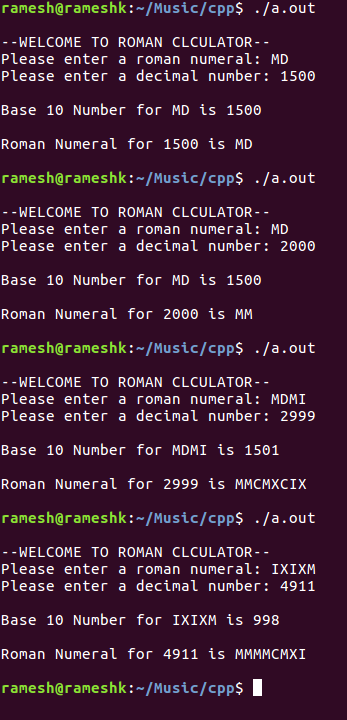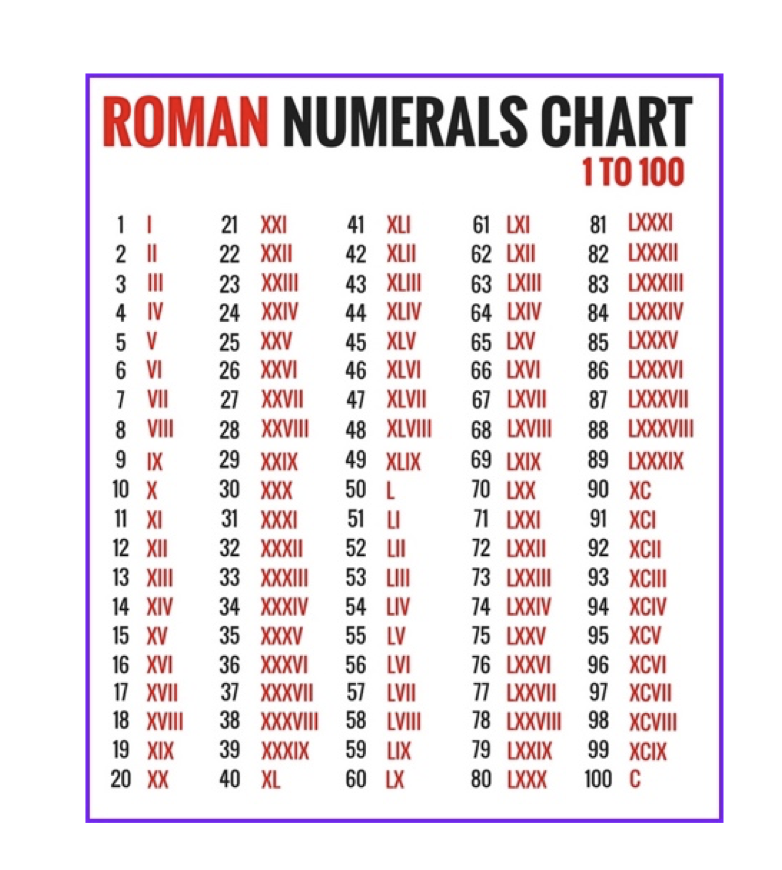# Lab Goal : The lab was designed to teach you more about objects and classes. Lab...

Lab Goal : The lab was designed to teach you more about objects and classes. Lab Description : In this program, you are to create a Roman Numeral class to handle roman numeral operations. How to convert a Roman Numeral to a standard base ten number : : Locate the first individual roman number in the roman number string. Sum up the numeric value of the individual number. Chop of the individual roman numeral from the string and continue the process if the string has more numbers left. How to convert a standard base ten number to a Roman Numeral : : Find the first Roman numeral less than the number. Add the roman numeral to a string. Subtract the value of the Roman Numeral from the number. Repeat this until the original number is less than the current Roman Numeral. Move to the next Roman Numeral in the list and repeat the process. Sample Data : see the main Sample Output : 10 is X 100 is C 1000 is M 2500 is MMD 1500 is MD 23 is XXIII 38 is XXXVIII 49 is XLIX LXXVII is 77 XLIX is 49 XX is 20 XLIX is 49 Roman 1 :: XXI Roman 2 :: XXV rOne.equals(rTwo) == false rOne.compareTo(rTwo) == -4 rTwo.compareTo(rOne) == 4

Hi Buddy,

I have Created a class with name as Roman, and in that i have created two class variables.

One variable is to store the roman numeral and the other is to store the base ten number.

And there are two function to convert the roman numeral to decimal and vice versa.

In the main program user need to enter a roman numeral and a decimal number, and these values will be assigned to the class variables by calling a constructor.

After that we will the function which are declared in the class, to perform the convertion.

Program:

#include<bits/stdc++.h>

#include<iostream>

using namespace std;

//class for roman numerals conversion

class Roman {

private:

//string to store the roman numeral

string romanString;

//int to store the decimal number

int num;

public:

//constructor to initialize the class varibales

Roman(string str, int n){

romanString=str;

num=n;

}

//function to convert the roman numeral to decimal value

void convertRoman() {

//map to store the roman and their corresponding values

map<char, int> roman;

roman['M'] = 1000;

roman['D'] = 500;

roman['C'] = 100;

roman['L'] = 50;

roman['X'] = 10;

roman['V'] = 5;

roman['I'] = 1;

//initializing the res value to 0

int res = 0;

for (int i = 0; i < romanString.size() - 1; ++i) {

if (roman[romanString[i]] < roman[romanString[i + 1]])

res -= roman[romanString[i]];

else

res += roman[romanString[i]];

}

res += roman[romanString[romanString.size() - 1]];

cout << "Base 10 Number for "<<romanString<<" is " << res << endl<<endl;

}

//function to convert decimal to roman numeral

void convertDecimal(){

cout << "Roman Numeral for "<<num<<" is ";

//STore the Roman values and their base values in arrays

int decimal[] = {1000,900,500,400,100,90,50,40,10,9,5,4,1};

char *symbol[] = {"M","CM","D","CD","C","XC","L","XL","X","IX","V","IV","I"};

int i = 0;

while(num){

//Initially which base value is going to divide this number will be the largest number

while(num/decimal[i]){

cout<<symbol[i];

//substract the largest base value from the number

num -= decimal[i];

}

i++;

}

cout<<endl<<endl;

}

};

//main function to call the functions declared in the

//Roman class

int main() {

string str;

int n;

cout<<"\n--WELCOME TO ROMAN CLCULATOR--"<<endl;

cout<<"Please enter a roman numeral: ";

cin>>str;

cout<<"Please enter a decimal number: ";

cin>>n;

cout<<endl;

Roman r(str,n);

r.convertRoman();

r.convertDecimal();

return 0;

}

/**

* 1.

*

--WELCOME TO ROMAN CLCULATOR--

Please enter a roman numeral: MD

Please enter a decimal number: 1500

Base 10 Number for MD is 1500

Roman Numeral for 1500 is MD

* 2.

--WELCOME TO ROMAN CLCULATOR--

Please enter a roman numeral: MD

Please enter a decimal number: 2000

Base 10 Number for MD is 1500

Roman Numeral for 2000 is MM

3.

--WELCOME TO ROMAN CLCULATOR--

Please enter a roman numeral: MDMI

Please enter a decimal number: 2999

Base 10 Number for MDMI is 1501

Roman Numeral for 2999 is MMCMXCIX

4.

--WELCOME TO ROMAN CLCULATOR--

Please enter a roman numeral: IXIXM

Please enter a decimal number: 4911

Base 10 Number for IXIXM is 998

Roman Numeral for 4911 is MMMMCMXI

*

*/

ouput screenshot:#### Earn Coin

Coins can be redeemed for fabulous gifts.Write a Python program (question2.py) that reads from a file called “input.txt” numbers in [1,39] separated in by commas. The numbers are in [1-99]. The program will then convert each number to a possible Roman Numeral equivalent, and print it on the screen. Remember, I is 1, V is 5, X is 10 For example, if the input is: 23, 11 the output is: XXIII, XI. ROMAN NUMERALS CHART 1 TO 100 69 LXIX 11 2 11 3 III 4...